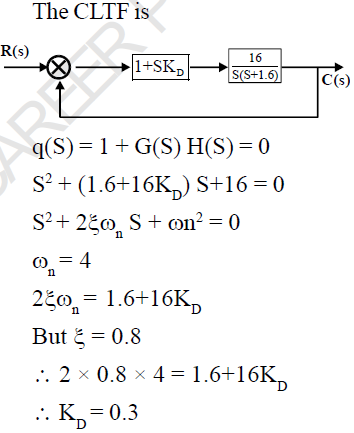Courses

# Test: Control System - 3

## 25 Questions MCQ Test GATE Electrical Engineering (EE) 2022 Mock Test Series | Test: Control System - 3

Description
This mock test of Test: Control System - 3 for GATE helps you for every GATE entrance exam. This contains 25 Multiple Choice Questions for GATE Test: Control System - 3 (mcq) to study with solutions a complete question bank. The solved questions answers in this Test: Control System - 3 quiz give you a good mix of easy questions and tough questions. GATE students definitely take this Test: Control System - 3 exercise for a better result in the exam. You can find other Test: Control System - 3 extra questions, long questions & short questions for GATE on EduRev as well by searching above.
QUESTION: 1

### The OLTF of closed loop system is given by the following Transfer function.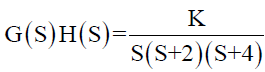The maximum value of K for which the system just become unstable is-

Solution: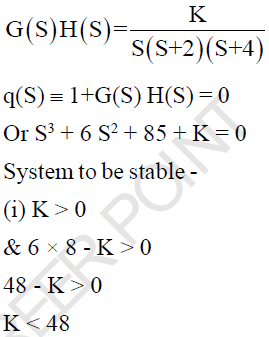QUESTION: 2

Solution:
QUESTION: 3

### The control system shown in the given fig has an internal rate Feedback loop. The closed-loop system for open and closed condition of the switch will be respectively-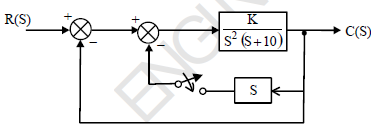Solution: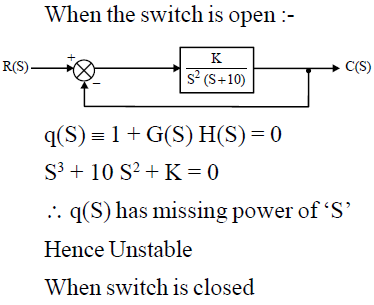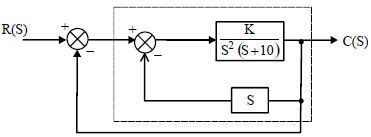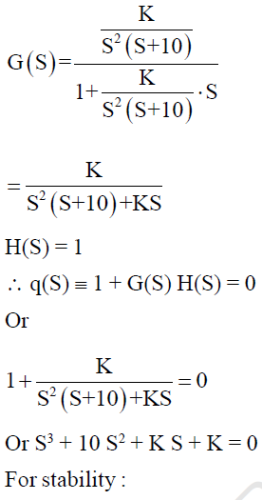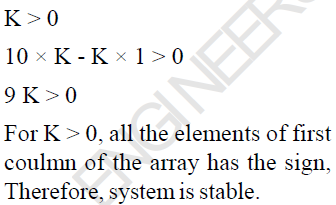*Answer can only contain numeric values
QUESTION: 4

An under damped second order  system having Transfer function of the form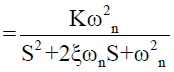has a frequency response shown below, The system gain ‘K’ is...?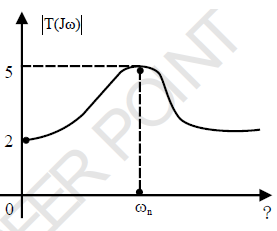Solution: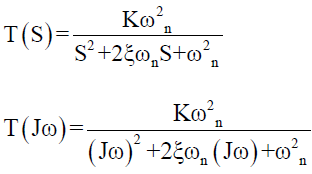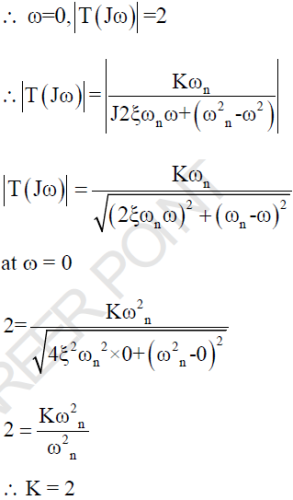QUESTION: 5

The OLTF of -ve UFB control system is given by-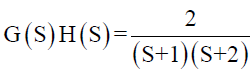The gain margin (in dB) is given by-

Solution: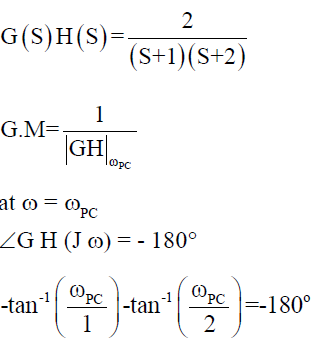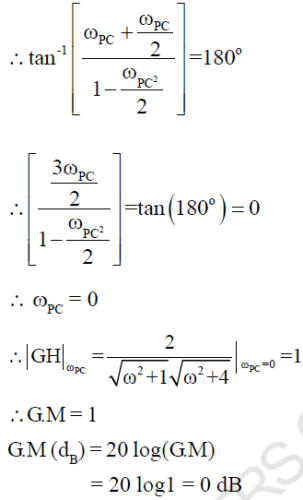QUESTION: 6

A unity Feed back system has forword loop gain T.F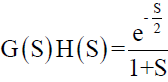. The cross over frequency (ωPC) is given by -

Solution: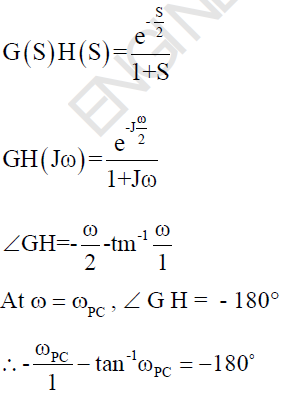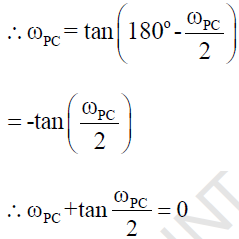QUESTION: 7

The Magnitude Bode Plot of a certain system is shown below.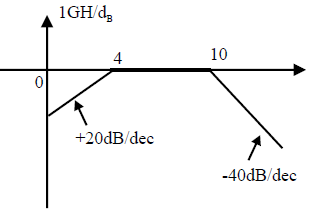What will be its Transfer function..?

Solution: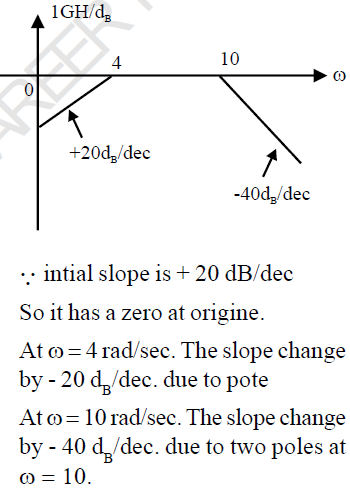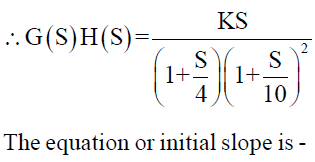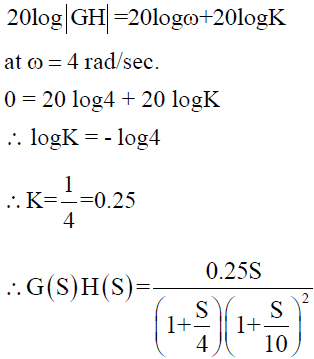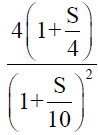QUESTION: 8

The T.F. of control system is given by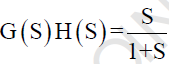. The polar plot of the system lies in-

Solution: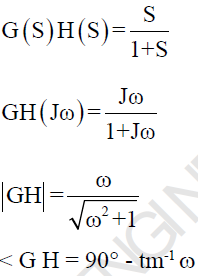QUESTION: 9

Which terminology deals with the excitation or stimulus applied to the system from an external source for the generation of an output?

Solution:

For the output of the system, mainly the input and state of the system are required and the excitation or stimulus applied to the system from an external source for the generation of the output is called input signal.

QUESTION: 10

The response C(t) of a system to an input r(t) is given by the following differential equation: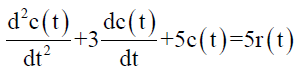The Transfer function of the system is given by-

Solution: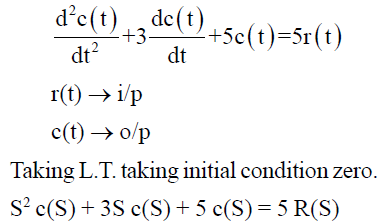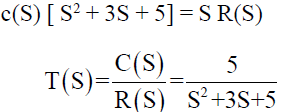QUESTION: 11

A second order system is defined by the following differential equation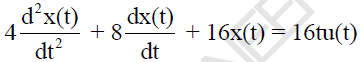The damping ratio and natural prog. For this system is respectively.

Solution: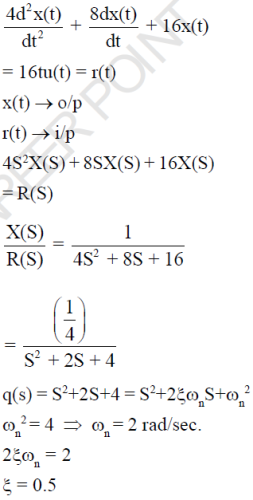QUESTION: 12

For the given system the magnitude of CLTF sensitivity w.r.t OLTF at  frequency ω = 1 rad/sec is ?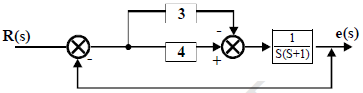Solution: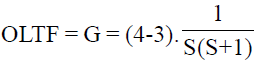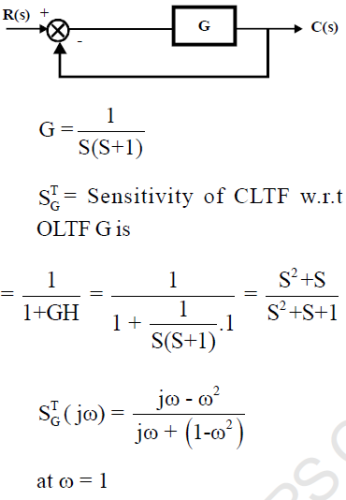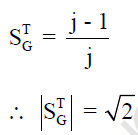QUESTION: 13

Find the impulse response of the system having transfer function each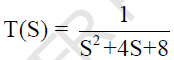Solution: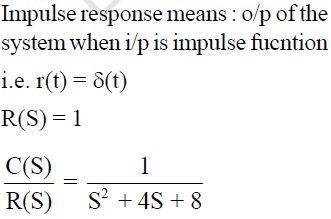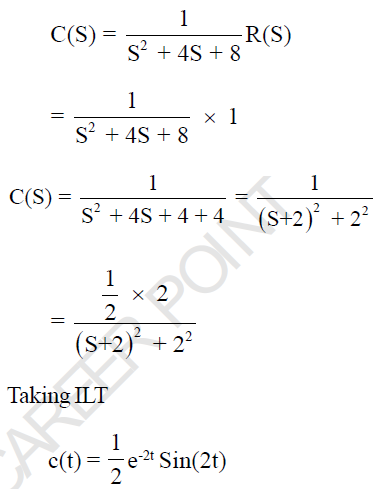QUESTION: 14

A closed loop control system has G(S) = 100/S2 and H(S) = (S+1) The steady state output for a unit step
input is…?

(Important - Enter only the numerical value in the answer)

Solution: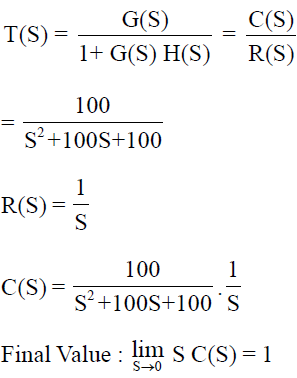QUESTION: 15

A linear time invariant system initially at rest, when subjected to unit step input gives a response y(t) = te-t, t > 0 The corresponding transfer function is

Solution: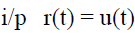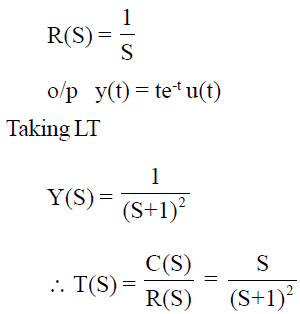QUESTION: 16

The responsse of a system is shown by the given wave-form. The correct root location in the S- Plane is ..?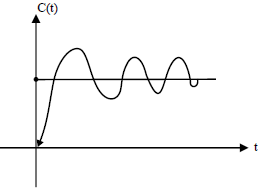Solution:

Given fig is response of the system which is under-damped

i.e. 0<ξ<1

For under-damped system, Poles are Comlex-Conjugate and lies on left half of S-Plane.

*Answer can only contain numeric values
QUESTION: 17

Consider a causal L T I system characterized by the Differential equations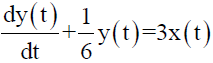The steady state o/p of the system to input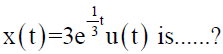(Important - Enter only the numerical value in the answer

Solution: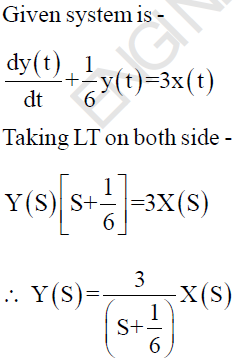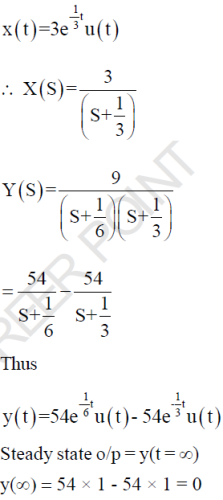QUESTION: 18

The system shown in the given fig. is subjected to a unit rump input.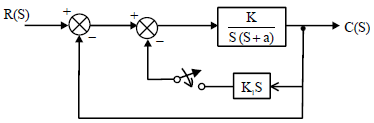On closing the switches

Solution: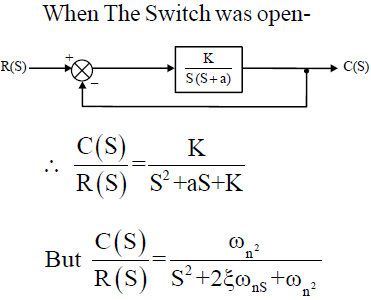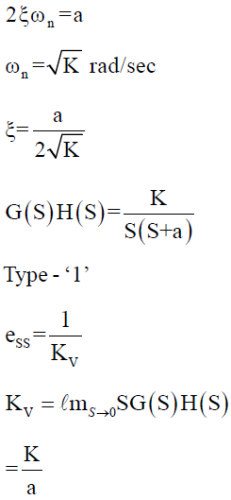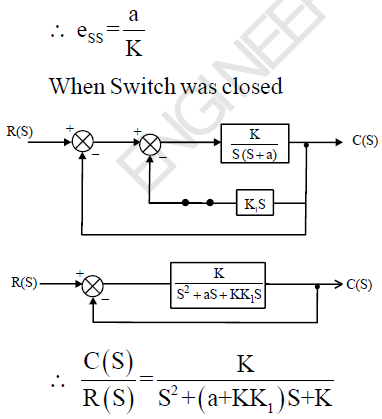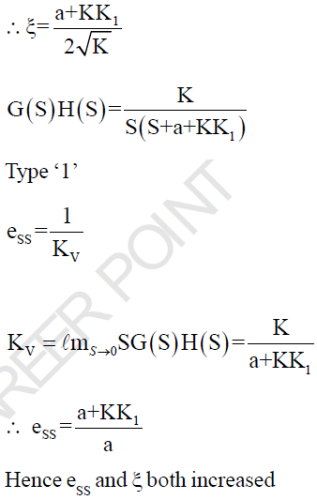QUESTION: 19

The impulse response of an initially relaxed linear system is e-2t , t > 0. To produce a response of t e- 2t , t >0, The input must be equal to -

Solution: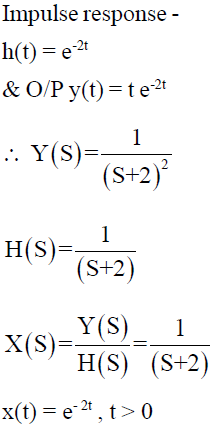QUESTION: 20

A control system is discribed by the following equation -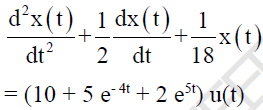The Natural time constants of the system are-

Solution: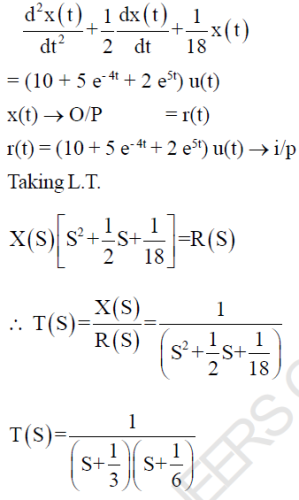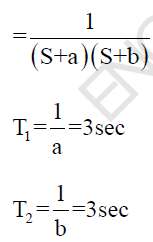QUESTION: 21

The root location for a second order system is shown, the dumping ratio and natural freq. of system is respectively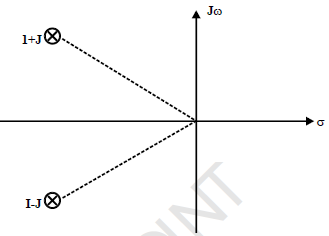Solution: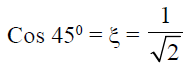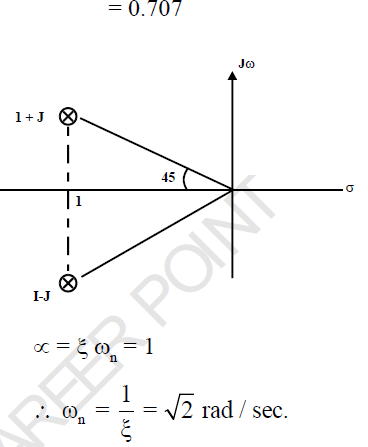QUESTION: 22

Consider UFB system with forward path T.F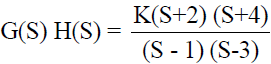The range of K for which system is stable

Solution: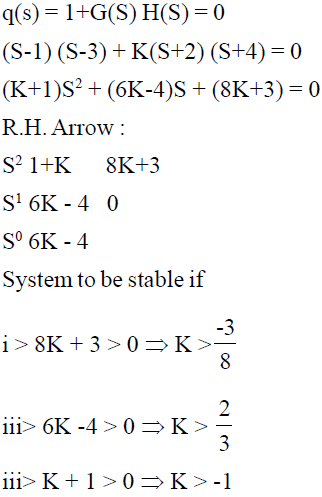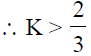*Answer can only contain numeric values
QUESTION: 23

An open loop control system results in a response of

(10e-2t - 3e-t) u(t)

For an unit impulse input. The D.C. gain of control system is….....?

(Important - Enter only the numerical value in the answer)

Solution: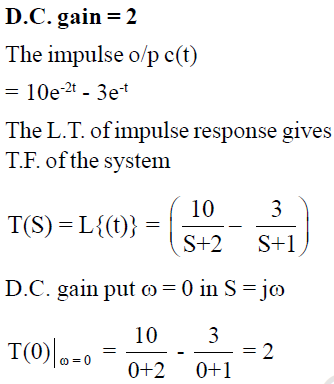QUESTION: 24

For the given system the value of  K and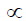so that the system oscillates at freq. ω = 1 rad/sec.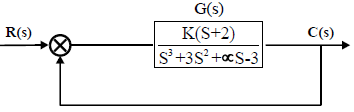(Important - Enter only the numerical value in the answer)

Solution:

K = 3 &= -2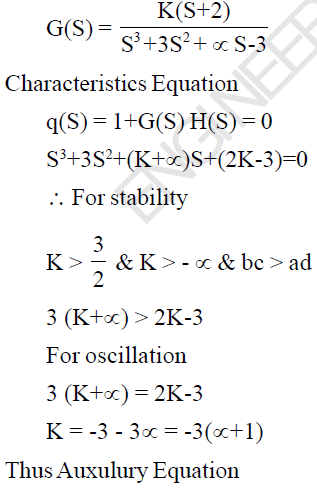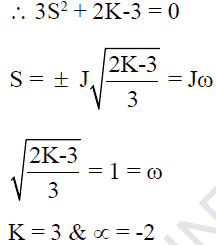*Answer can only contain numeric values
QUESTION: 25

A system with derivative controller is given as below. The value of KD for which the damping ratio becomes 0.8 is ?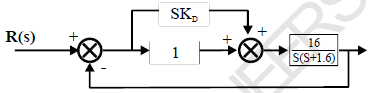(Important - Enter only the numerical value in the answer)

Solution: